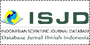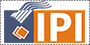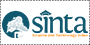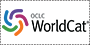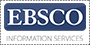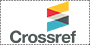Comparison of Estimated Glomerular Filtration Rate Mean Value of HARUS 15-30-60, HADI, and ASIAN Fomula Accuracy in Diabetes Mellitus Type 2

Sylvia Rachmayati, Ida Parwati, Abdul Hadi Martakusumah, Rachmat Soelaeman, Nanan Sekarwana

Abstract

Objective: To compare the accuracy of HARUS 15-30-60, HADI, and Asian Formulas (Chinese-equation (Ch-E), Japanese-equation (Jp-E), and Thai- equation (Th-E)) for estimated glomerular filtration rate (eGFR).

Methods: The Kidney Dialysis Outcome Quality Initiative (KDOQI) has published a guideline to measure renal function, which is based on glomerular filtration rate (GFR). This procedure is complicated and expensive, therefore an estimated GFR (eGFR) has been proposed. The modification of diet in renal disease (MDRD) study prediction equation is the most frequently eGFR used. This method still have a weakness in accuracy, so the chronic kidney disease epidemiology collaboration (CKD-EPI) formula is developed. Since CKD-EPI is not practical for daily use, the MDRD is published for Asian population that includes Ch-E, Jp-E, and Th-E. In Indonesia, the MDRD formula has not been validated using any gold standard, therefore 2 new formulas have been developed, i.e. HARUS 15-30-60 and HADI formulas. In this study, we analyzed 102 medical records of Diabetes Mellitus Type 2 (DMT2) patients who visited Dr. Hasan Sadikin General Hospital, Bandung during the period of 2012 to 2013. We analyzed the data using HARUS 15-30-60, HADI, Asian formulas, and then compared them to CKD-EPI to see the accuracy. Statistical analysis used was paired t-test in SPSS-17 program.

Results: The accuracy of the different formulas are as follows: HADI (p=0.173), HARUS 15-30-60 (p=0.060), Ch-E (p=0.001), Th-E (p=0.000), and Jp-E (p=0.000).

Conclusions: HADI is the most accurate formula, followed by HARUS Formula, Ch-E, and Th-E and Jp-E, respectively.

Keywords: Chinese-equation, HADI and HARUS 15-30-60 formulas, Japanese-equation, and Thai-equation

Keywords

Chinese-equation, HADI and HARUS 15-30-60 formulas, Japanese-equation, and Thai-equation

Full Text:

PDF

This journal indexed by: